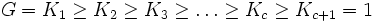# Strongly central series

This article defines a property that can be evaluated for a subgroup series

View a complete list of properties of subgroup series

## Definition$G = K_1 \ge K_2 \ge K_3 \ge \ldots \ge K_c \ge K_{c+1} = 1$

is termed strongly central if$[K_i,K_j] \le K_{i+j}$ for every$i,j = 1,2,\ldots,c$.

(A similar definition works for transfinite series).

## Examples

The lower central series and upper central series of a nilpotent group are both examples of strongly central series. Further information: Lower central series is strongly central, Upper central series is strongly central